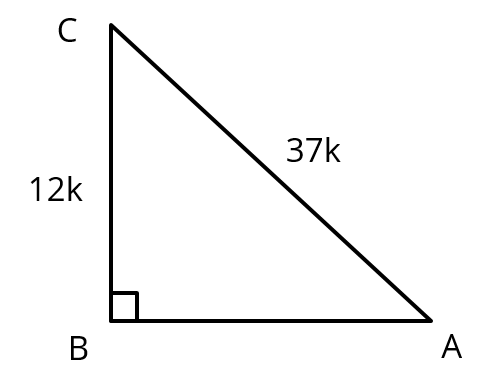# Extra Questions Class 10 Maths Chapter 8 #2

#### Trigonometry | Trigonometric Values

This CBSE class 10 Maths practice question is from the topic Trigonometry. It tests the concept of computing trigonometric values if the sides of a right triangle are given. We will use Pythagoras Theorem to compute all sides of a right triangle. Another trigonometry extra question that helps you learn a new Pythagorean Triplet. Questions of this type are most likely to be tested as multiple choice questions in Class 10 board exam.

Question 2: Given sin A = $$frac{12}{37}$, find cos A and tan A. ## Target Centum in CBSE 10th Maths #### Online CBSE Course online.maxtute.com ### Video Explanation ## NCERT Solution to Class 10 Maths #### With Videos ### Explanatory Answer | Trigonometry Important Question 2sin A = $\frac{\text{side opposite to ∠A}}{\text{hypotenuse}}$ = $\frac{\text{BC}}{\text{AC}}$= $\frac{12}{37}$ ∴ If BC = 12k, AC = 37k Using Pythagoras theorem AB = $\sqrt{AC^2− BC^2}$ $AB$ = $\sqrt{$37k$^2−(12k)^2}) = $\sqrt{1369k^2 − 144k^2 }$ = $\sqrt{1225k^2}$= $35k$

cos A = $\frac{\text{side adjacent to ∠A}}{\text{hypotenuse}}$ = $\frac{\text{AB}}{\text{AC}}$ = $\frac{\text{35k}}{\text{37k}}$ = $\frac{\text{35}}{\text{37}}$
tan A = $\frac{\text{side opposite to ∠A}}{\text{side opposite to ∠A}}$ = $\frac{\text{BC}}{\text{AB}}$ = $\frac{\text{12k}}{\text{35k}}$ = $\frac{\text{12}}{\text{35}}$

#### Try CBSE Online CoachingClass 10 Maths

Register in 2 easy steps and
Start learning in 5 minutes!